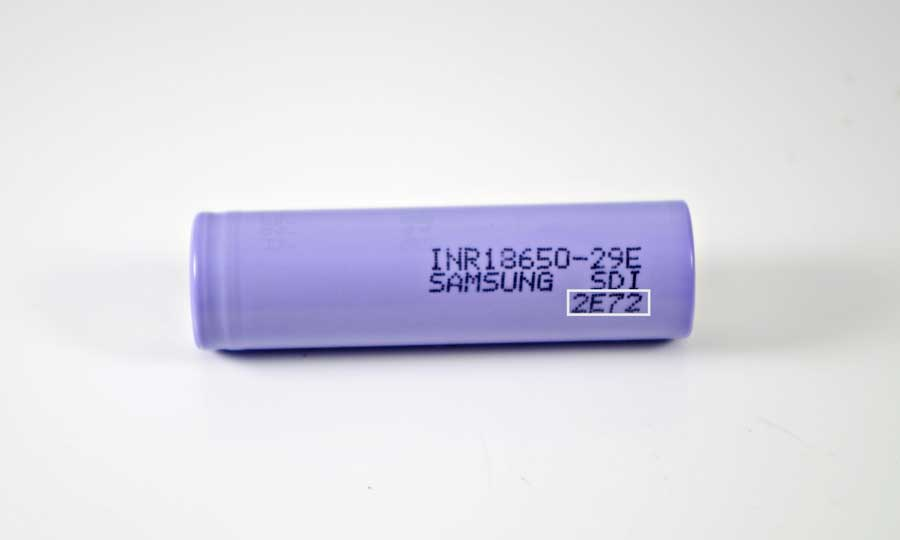# Part 1- Recognize Samsung 18650 Battery Manufacture Date Code

It’s very easy to recognize the date code early Samsung 18650 batteries. There’ re 3 lines on the battery body and the third line is the date code.The first digit = Year, the second digit = Month, and the third digit = Week.However, from the year of 2010, Samsung changed its code rules. Moreover, Samsung lithium batteries are divided into SDI ( are manufactured in Korea) and SDIEM ( are manufactured in Malaysia). And the date code rules of SDI and SDIEM are different.

The battery below is from SDI, and on the second line, we can see “SDI”.On the third line of the PVC, there’ re 4 digits.

1. The first digit = Version
2. The second digit = Year ( A = 2010, B = 2011, C = 2012, D = 2013, E = 2014, F = 2015).
3. The third digit = Month (1, 2, 3…..9, A= 10, B = 11, C = 12).
4. The fourth digit may mean Day, not so sure. It seems that, 3 means the day of 03, 13, and 30 at the same time.

So can be figured out that it was manufactured in July, 2014.

The battery below is from SDIEM, and also has 4 digits.1. The first digit = Version
2. The second digit = Year ( A = 2010, B = 2011, C = 2012, D = 2013, E = 2014, F = 2015).
3. The third digit = Month ( O = 1, P = 2, Q = 3, R = 4, S = 5, T = 6, U = 7, V = 8, W = 9, X = 10, Y = 11, Z = 12).
4. The fourth digit may mean Day, not so sure. It seems that, 3 means the day of 03, 13, and 30 at the same time.

Therefore it was made in May, 2013.

1.Jamie Yavis on

Thanks for this information – i wish i had found your page 4 hours earlier; i had been searching for the line 3 meaning, thinking it was possibly a series identification; but a date, and place of manufacturing is a large bonus to know!!